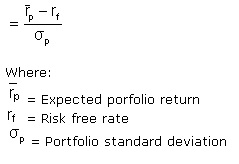# Sharpe Ratio

Posted in Finance, Accounting and Economics Terms, Total Reads: 1496
Advertisements

## Definition: Sharpe Ratio

This is a ratio developed and named after the great Nobel laureate William F Sharpe in 1966. It measures the risk adjusted performance. The ratio is calculated as by subtracting risk free rate (determined as the rate on 10 year US treasury bonds) from the rate of return on the portfolio and dividing this figure by the standard deviation of the portfolio.This is the ex-ante formula for the ratio. The ex-post formula is similar except instead of the expected rate of return it uses the realized rate of return.

This ratio signifies whether the returns on a given portfolio are due to excessive risk or actual smart investment decisions. As a portfolio might generate higher returns viz a viz its peers but it is a good investment only when these higher returns come with lower risk i.e. lower standard deviation of the portfolio. The higher the Sharpe ratio, the better is the risk adjusted performance of that portfolio. A Sharpe ratio with value less than 1 implies that the return is lower than risk free rate and hence a risk less asses would be better alternative.

Hence, this concludes the definition of Sharpe Ratio along with its overview.

Advertisements

Browse the definition and meaning of more terms similar to Sharpe Ratio. The Management Dictionary covers over 7000 business concepts from 6 categories.

Search & Explore : Management Dictionary

Similar Definitions from same Category: